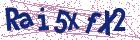# A parametric iteration method for solving Lane-Emden type equations

Document Type : Research Article

Author

Department of Basic Sciences, Jundi-Shapur University of Technology, Dezful, Iran.

Abstract

In this paper, an analytical method called the parametric iteration method (PIM) is presented for solving the second-order singular IVPs of Lane-Emden type, and its local convergence is discussed. Since it is often useful to have an approximate analytical solution to describe the Lane-Emden type equa- tions, especially for ones where the closed-form solutions do not exist at all, therefore, an eﬀective improvement of the PIM is further proposed that is ca- pable of obtaining an approximate analytical solution. The improved PIM is ﬁnally treated as an algorithm in a sequence of intervals for ﬁnding accurate approximate solutions of the nonlinear Lane-Emden type equations. Also, we show how to identify an approximate optimal value of the convergence accelerating parameter within the frame of the method. Some examples are given to demonstrate the eﬃciency and accuracy of the proposed method.

Keywords20.1001.1.24236977.2017.7.1.3.2

#### References

1. Bender, C.M., Milton, K.A., Pinsky, S.S., and Simmons L.M. A new per turbative approach to nonlinear problems, J Math Phys. 30 (1989) 1447-1455.
2. Chandrasekhar, S. Introduction to the Study of Stellar Structure, Dover, New York, 1967.
3. Chowdhury, M.SH., and Hashim, I. Solutions of a class of singular second order IVPs by homotopy-perturbation method, Phys. Lett. A 365 (2007)439-447.
4. Davis, H.T. Introduction to Nonlinar Differential and Integral Equations, Dover, New York, 1962.
5. Ghorbani, A. Toward a new analytical method for solving nonlinear fractional differential equations, Comput. Methods Appl. Mech. Engrg. 197 (2008) 4173-4179.
6. Ghorbani, A., and Saberi-Nadjafi, J. A piecewise-spectral parametric it eration method for solving the nonlinear chaotic Genesio system, Math. Comput. Modelling 54 (2011) 131-139.
7. He, J.H. Variational approach to the Lane-Emden equation, Appl Math Comput 143 (2003) 539-541.
8. Hosseini, S. Gh., and Abbasbandy S. Solution of Lane-Emden Type Equa tions by Combination of the Spectral Method and Adomian Decomposi tion Method, Mathematical Problems in Engineering, vol. 2015, Article ID 534754, 10 pages, 2015. doi:10.1155/2015/534754.
9. Krivec, R., and Mandelzweig V.B. Numerical investigation of quasilin earization method in quantum mechanics, Comput Phys Commun 138 (2001) 69-79.
10. Liao, SH.J. A new analytic algorithm of Lane-Emden type equations, Appl Math Comput 142 (2003) 1-16.
11. Mandelzweig, V.B., and Tabakin, F. Quasilinearization approach to nonlinear problems in physics with application to nonlinear ODEs, Comput Phys Commun. 141 (2001) 268-281.
12. Ramos, J.I. Linearization techniques for singular initial-value problems of ordinary differential equations, Appl. Math. Comput. 161 (2005) 525-542.
13. Ramos, J.I. Series approach to the Lane-Emden equation and comparison with the homotopy perturbation method, Chaos Solitons Fractals 38 (2008) 400-408.
14. Richardson, O.U. The Emission of Electricity from Hot Bodies, London, 1921.
15. Saberi-Nadjafi, J., and Ghorbani, A. Piecewise-truncated parametric iteration method: a promising analytical method for solving Abel differential equations, Z. Naturforsch. 65a (2010) 529-539.
16. Shawagfeh, N.T. Nonperturbative approximate solution for Lane-Emden equation, J.Math. Phys. 34 (1993) 4364-4369.
17. Wazwaz, A.M. A new algorithm for solving differential equations of LaneEmden type, Appl. Math. Comput. 118 (2001) 287-310.
18. Yildirim, A., and Ozis, T. ¨ Solutions of singular IVPs of Lane-Emden type by homotopy perturbation method, Physics Letters A 369 (2007) 70-76.